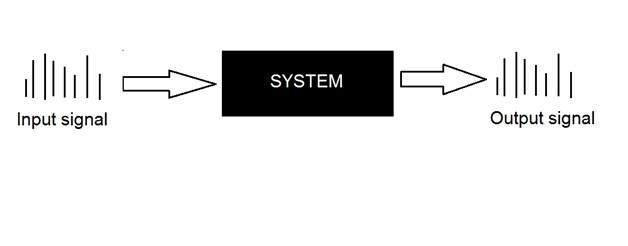## Signals

In electrical AND electronics engineering, the fundamental quantity of representing some information is called a signal. It does not matter what the information is i-e: Analog or digital information. In mathematics, a signal is a function that conveys some information. In fact any quantity measurable through time over space or any higher dimension can be taken as a signal. A signal could be of any dimension and could be of any form.

## Analog signals

A signal could be an analog quantity that means it is defined with respect to the time. It is a continuous signal. These signals are defined over continuous independent variables. They are difficult to analyze, as they carry a huge number of values. They are very much accurate due to a large sample of values. In order to store these signals , you require an infinite memory because it can achieve infinite values on a real line. Analog signals are denoted by sin waves.

For example:

## Human voice

Human voice is an example of analog signals. When you speak, the voice that is produced travel through air in the form of pressure waves and thus belongs to a mathematical function, having independent variables of space and time and a value corresponding to air pressure.

Another example is of sin wave which is shown in the figure below.

Y = sin(x) where x is independent## Digital signals

As compared to analog signals, digital signals are very easy to analyze. They are discontinuous signals. They are the appropriation of analog signals.

The word digital stands for discrete values and hence it means that they use specific values to represent any information. In digital signal, only two values are used to represent something i-e: 1 and 0 (binary values). Digital signals are less accurate then analog signals because they are the discrete samples of an analog signal taken over some period of time. However digital signals are not subject to noise. So they last long and are easy to interpret. Digital signals are denoted by square waves.

For example:

## Computer keyboard

Whenever a key is pressed from the keyboard, the appropriate electrical signal is sent to keyboard controller containing the ASCII value that particular key. For example the electrical signal that is generated when keyboard key a is pressed, carry information of digit 97 in the form of 0 and 1, which is the ASCII value of character a.

## Difference between analog and digital signals

Comparison element Analog signal Digital signal
Analysis Difficult Possible to analyze
Representation Continuous Discontinuous
Accuracy More accurate Less accurate
Storage Infinite memory Easily stored
Subject to Noise Yes No
Recording Technique Original signal is preserved Samples of the signal are taken and preserved
Examples Human voice, Thermometer, Analog phones e.t.c Computers, Digital Phones, Digital pens, e.t.c

## Systems

A system is a defined by the type of input and output it deals with. Since we are dealing with signals, so in our case, our system would be a mathematical model, a piece of code/software, or a physical device, or a black box whose input is a signal and it performs some processing on that signal, and the output is a signal. The input is known as excitation and the output is known as response.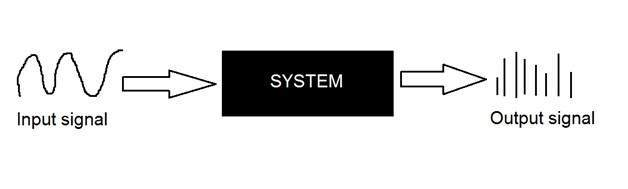In the above figure a system has been shown whose input and output both are signals but the input is an analog signal. And the output is an digital signal. It means our system is actually a conversion system that converts analog signals to digital signals.

## Conversion of analog to digital signals

Since there are lot of concepts related to this analog to digital conversion and vice-versa. We will only discuss those which are related to digital image processing.There are two main concepts that are involved in the coversion.

• Sampling
• Quantization

### Sampling

Sampling as its name suggests can be defined as take samples. Take samples of a digital signal over x axis. Sampling is done on an independent variable. In case of this mathematical equation: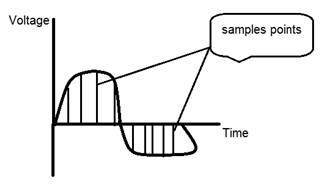Sampling is done on the x variable. We can also say that the conversion of x axis (infinite values) to digital is done under sampling.

Sampling is further divide into up sampling and down sampling. If the range of values on x-axis are less then we will increase the sample of values. This is known as up sampling and its vice versa is known as down sampling.

### Quantization

Quantization as its name suggest can be defined as dividing into quanta (partitions). Quantization is done on dependent variable. It is opposite to sampling.

In case of this mathematical equation y = sin(x)

Quantization is done on the Y variable. It is done on the y axis. The conversion of y axis infinite values to 1, 0, -1 (or any other level) is known as Quantization.

These are the two basics steps that are involved while converting an analog signal to a digital signal.

The quantization of a signal has been shown in the figure below.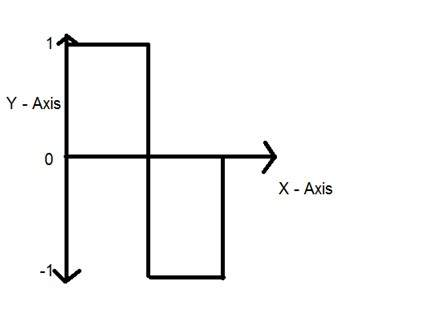## Why do we need to convert an analog signal to digital signal.

The first and obvious reason is that digital image processing deals with digital images, that are digital signals. So when ever the image is captured, it is converted into digital format and then it is processed.

The second and important reason is, that in order to perform operations on an analog signal with a digital computer, you have to store that analog signal in the computer. And in order to store an analog signal, infinite memory is required to store it. And since thats not possible, so thats why we convert that signal into digital format and then store it in digital computer and then performs operations on it.

## Continuous systems vs discrete systems

### Continuous systems

The type of systems whose input and output both are continuous signals or analog signals are called continuous systems.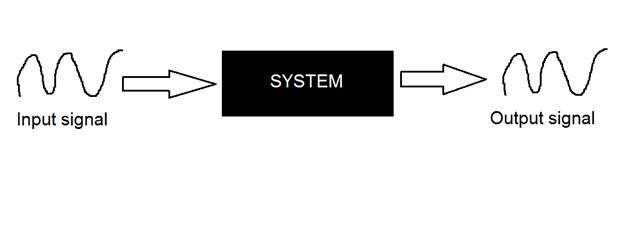### Discrete systems

The type of systems whose input and output both are discrete signals or digital signals are called digital systems.